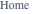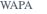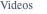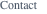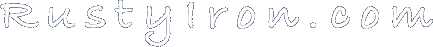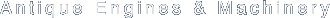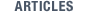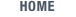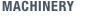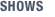Reproduced from Loetzer's Hand-book, 9th Edition, 1913

Gasoline engines are known as internal combustion engines and are divided into two general classes, namely two-cycle and four-cycle engines. A cycle represents one stroke of the piston or one-half revolution of the crank-shaft, a complete revolution therefore represents two cycles. In a two-cycle engine the power impulse occurs at each revolution, while in a four-cycle engine it occurs at every other revolution, hence the terms two and four-cycle engines.

Both classes have their own particular advantages and uses. For automobiles and stationary uses the four-cycle is most employed, whereas, for motor boat use the two-cycle has the preference.

The horse power is designated both as indicated horse power, also as brake test horse power. The I.H.P. is the theoretical H.P. which is found by figuring certain formulas, in which the diameter of the bore, the length of stroke in inches, and the number of revolutions per minute form the basis for the calculation. The results found by the use of these formulas indicate, as the term implies, the number of H.P. which that engine is supposed to be able to develop.

The B.T.H.P. is the power the engine actually develops in service, and is considerable less than the I.H.P. This depends entirely upon the degree of mechanical perfection attained in the construction of same. If the construction and compression are good the engine may reach an efficiency of from 80 to 88 per cent. of the I.H.P.

Owing to the great number of factors which influence the power delivered by high-speed internal combustion engines, the only reliable way to definitely determine the H.P. they actually furnish is by the brake test.

For the purpose of comparison and quick calculation, however, a number of useful formulas have been compiled and are generally used by both manufacturers and buyers to find the I.H.P. Following is one of the simplest and most popular.

DETERMINING THE HORSE POWER OF AN ENGINE.

Multiply the area of the cylinder by the length of stroke in inches and that product by the number of revolutions per minute then divide by the constant 10000 for two-cycle or 13400 for four-cycle engine, the result will be the I.H.P.

EXAMPLE

Four-cycle engine, 4" bore, 4" stroke, 1000 revolutions per minute, constant 13400. Then: 4" bore equals 12.56 area, then 12.56 x 4 equals 50.24 x 1000 equals 50240 divided by 13400 equals 3¾ I.H.P. for one cylinder, then to find the combined H.P. for multi- cylinder engine, multiply by the number of cylinders.